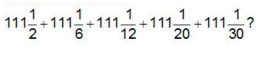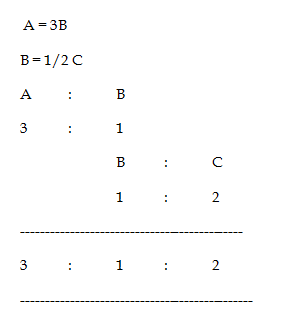# SSC CGL 2018 Practice Test Papers | Quantitative Aptitude (Day-56)

Dear Aspirants, Here we have given the Important SSC CGL Exam 2018 Practice Test Papers. Candidates those who are preparing for SSC CGL 2018 can practice these questions to get more confidence to Crack SSC CGL 2018 Examination.

[WpProQuiz 3678]

Click “Start Quiz” to attend these Questions and view Explanation

1) How many prime numbers are there from 1 to 40?

a) 10

b) 11

c) 12

d) 13

2) What is the value of 1.44 × 0.02 + 2.2 × 0.01?

a) 0.502

b) 0.508

c) 0.0502

d) 0.0508

3) What is the value of 75% of 50% of 1/3of 3600?

a) 450

b) 300

c) 600

d) 750

4) Simple and compound interest on a principal at a certain rate of interest for 2 years is Rs 1400 and Rs 1470 respectively. What is the principal (in Rs)?

a) 8000

b) 6000

c) 7000

d) 5000

5) If cost price of 12 articles is equals to selling price of 15 articles, then what will be the profit/ loss percentage?

a) 33 1/3 %

b) 25%

c) 20%

d) 18 2/3 %

6) A man is walking at a speed of 8 kmph. After every km, he takes a rest of 6 minutes. How much time (in minutes) will he take to cover a distance of 8 kms?

a) 100 minutes

b) 102 minutes

c) 106 minutes

d) 108 minutes

7) A, B and C started a business together A’s capital is thrice of B’s capital and B’s capital is half of C’s Capital. What is the respective ratio of their capitals?

a) 6: 3: 2

b) 6: 3: 1

c) 2: 3: 6

d) 3: 1: 2

8) What is the value ofa) 111 5/6

b) 111 1/6

c) 555 1/6

d) 555 5/6

9) A, B and C together can complete a work in 20 days. A and C together can complete the work in 70 days. Then B alone can complete the same work in many days?

a) 36 days

b) 72 days

c) 28 days

d) 42 days

10) The ratio of speed of Ravi, Pavi, and Kavi is 2 : 3 : 4 respectively. What is the ratio of the time taken by Ravi, Pavi and kavi to cover same distance?

a) 4: 3: 6

b) 4: 3: 2

c) 1: 3: 6

d) 6: 4: 3

Prime numbers 1 to 40: 2, 3, 5, 7, 11, 13, 17, 19, 23, 29, 31 and 37

1.44 × 0.02 + 2.2 × 0.01 = 0.0508

75% of 50% of 1/3 of 3600 = 3/4 × 1/2 × 1/3 × 3600 = 450

Simple interest for 2 years = 1400

1. I for one year = 700

C.I – S. I = 1470 – 1400 = 70

70/700 * 100 = 10%

10 % ———- 700

100 % ——– 7000

Principal= 7000

C.P of 12 = S.P of 15

3/12 * 100 = 100 /4 = 25% loss

Speed = 8 kmph

Distance = 8 km

Time = Distance / Speed = 8 km / 8 kmph = 1 hour = 60 minutes

In 8 km he take rest is 7 times = 7 * 6 = 42 minutes

Total time taken = 60 + 42 = 102 minutes111 ½ + 111 1/6 + 111 1/12 + 111 1/20 + 111 1/30

= 111 + 111 + 111 + 111 + 111 (1/2 + 1/6 + 1/12 + 1/20 + 1/30)

= 555 (50/60) = > 555   5/6

A+B+C = 1/ 20

A+C = 1/70

B + 1/70 = 1/20

B = 1/ 20 – 1/70

B = (7 – 2)/ 140 = 5 / 140 = 28 days

Time inversely proportional to Speed

Speed = 2 : 3: 4

Time = 1/2 : 1/3 : 1/4

Time = 6: 4: 3